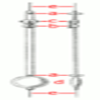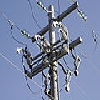Search by Topic

Resources tagged with 1st and 2nd order linear differential equations with constant coefficients similar to The Not-so-simple Pendulum 2:

Filter by: Content type:
Age range:
Challenge level:

There are 5 results

Broad Topics > Calculus > 1st and 2nd order linear differential equations with constant coefficientsThe Not-so-simple Pendulum 2

Age 16 to 18 Challenge Level:

Things are roughened up and friction is now added to the approximate simple pendulumThe Real Hydrogen Atom

Age 16 to 18 Challenge Level:

Dip your toe into the world of quantum mechanics by looking at the Schrodinger equation for hydrogen atomsDifferential Equation Matcher

Age 16 to 18 Challenge Level:

Match the descriptions of physical processes to these differential equations.Differential Electricity

Age 16 to 18 Challenge Level:

As a capacitor discharges, its charge changes continuously. Find the differential equation governing this variation.Phase Space

Age 16 to 18 Challenge Level:

Explore the issues surrounding the concept of Phase Space.